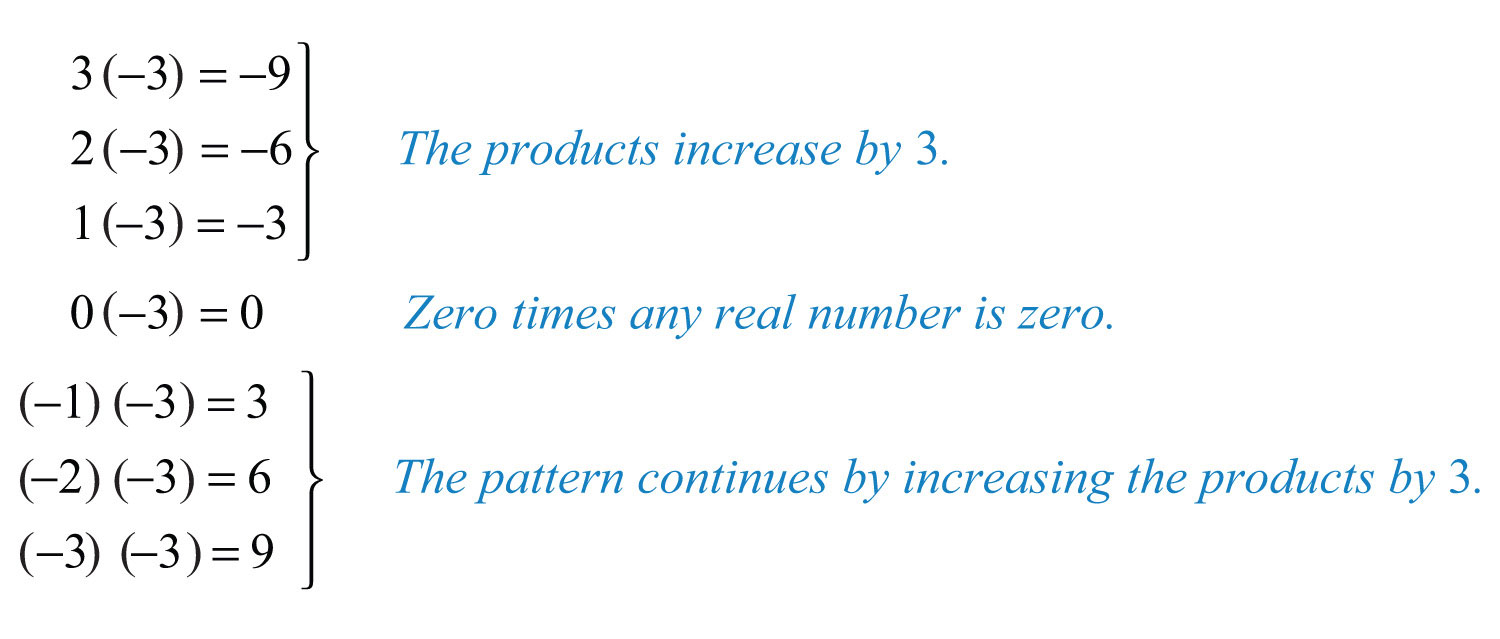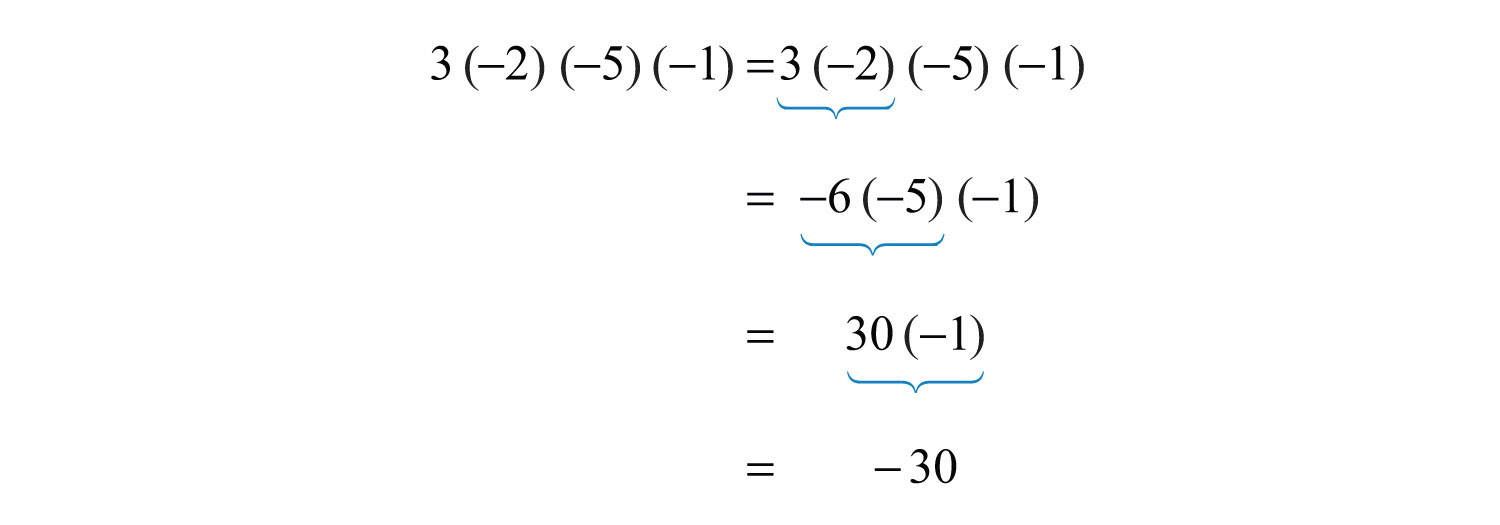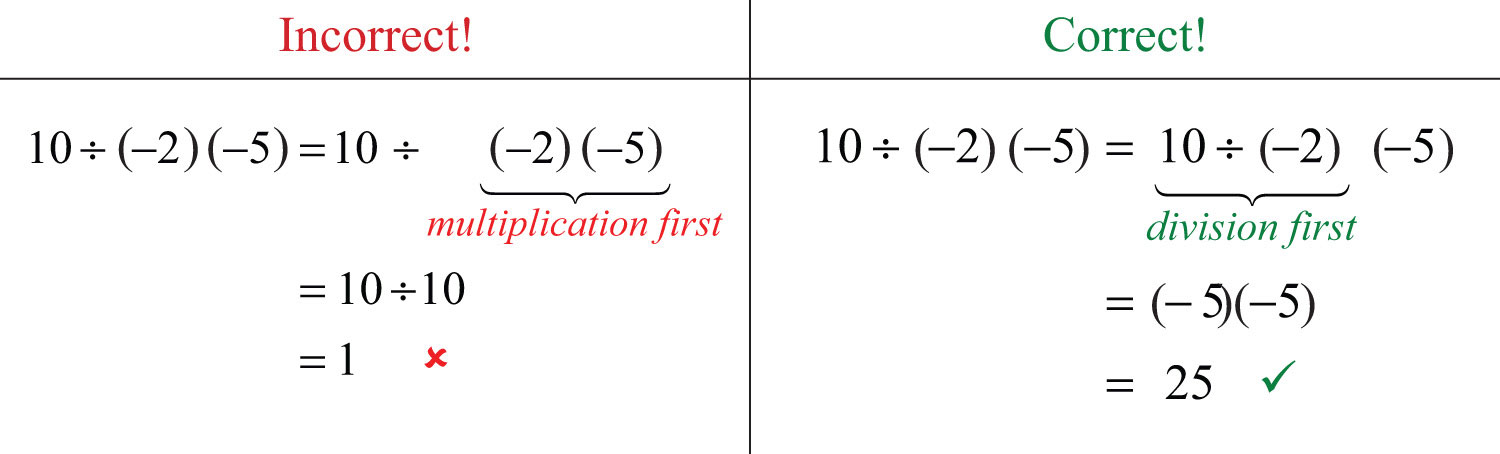This is “Multiplying and Dividing Integers”, section 1.3 from the book Beginning Algebra (v. 1.0). For details on it (including licensing), click here.

Has this book helped you? Consider passing it on:
Creative Commons supports free culture from music to education. Their licenses helped make this book available to you.
DonorsChoose.org helps people like you help teachers fund their classroom projects, from art supplies to books to calculators.

## 1.3 Multiplying and Dividing Integers

### Learning Objectives

1. Multiply and divide signed integers.
2. Translate English sentences involving multiplication and division into mathematical statements.
3. Determine the prime factorization of composite numbers.
4. Interpret the results of quotients involving zero.

## Multiplication and Division

We begin with a review of what it means to multiply and divide signed numbers. The result of multiplying real numbers is called the productThe result of multiplying. and the result of dividing is called the quotientThe result after dividing.. Recall that multiplication is equivalent to adding:Clearly, the product of two positive numbers is positive. Similarly, the product of a positive number and negative number can be written as shown:We see that the product of a positive number and a negative number is negative. Next, explore the results of multiplying two negative numbers. Consider the products in the following illustration and try to identify the pattern:This shows that the product of two negative numbers is positive. To summarize,The rules for division are the same because division can always be rewritten as multiplication:The rules for multiplication and division should not be confused with the fact that the sum of two negative numbers is negative.

Example 1: Simplify:

a. $(−3)+(−5)$

b. $(−3)(−5)$

Solution: Here we add and multiply the same two negative numbers.

a. The result of adding two negative numbers is negative.b. The result of multiplying two negative numbers is positive.Given any real numbers a, b, and c, we have the following properties of multiplication:

 Zero factor propertyGiven any real number a, $a⋅0=0⋅a=0.$: $a⋅0=0⋅a=0$ Multiplicative identity propertyGiven any real number a, $a⋅1=1⋅a=a.$: $a⋅1=1⋅a=a$ Associative propertyGiven any real numbers a, b, and c, $(a⋅b)⋅c=a⋅(b⋅c).$: $(a⋅b)⋅c=a⋅(b⋅c)$ Commutative propertyGiven any real numbers a and b, $a⋅b=b⋅a.$: $a⋅b=b⋅a$

Example 2: Simplify:

a. $5⋅0$

b. $10⋅1$

Solution:

a. Multiplying by zero results in zero.b. Multiplying any real number by one results in the same real number.Example 3: Simplify:

a. $(3⋅7)⋅2$

b. $3⋅(7⋅2)$

Solution:

a.b.The value of each expression is 42. Changing the grouping of the numbers does not change the result.At this point, we highlight that multiplication is commutative: the order in which we multiply does not matter and yields the same result.On the other hand, division is not commutative.Use these properties to perform sequential operations involving multiplication and division. When doing so, it is important to perform these operations in order from left to right.

Example 4: Simplify: $3(−2)(−5)(−1)$.

Solution: Multiply two numbers at a time as follows:Because multiplication is commutative, the order in which we multiply does not affect the final answer. When sequential operations involve multiplication and division, order does matter; hence we must work the operations from left to right to obtain a correct result.

Example 5: Simplify: $10÷(−2)(−5)$.

Solution: Perform the division first; otherwise, the result will be incorrect.Notice that the order in which we multiply and divide does affect the final result. Therefore, it is important to perform the operations of multiplication and division as they appear from left to right.

Example 6: Simplify: $−6(3)÷(−2)(−3)$.

Solution: Work the operations one at a time from left to right.Try this! Simplify: $−5÷5⋅2(−3)$.

### Video Solution

(click to see video)

Within text-based applications, the symbol used for multiplication is the asteriskThe symbol (*) that indicates multiplication within text-based applications. (*) and the symbol used for division is the forward slash (/).The set of even integersIntegers that are divisible by two or are multiples of two. is the set of all integers that are evenly divisible by 2. We can also obtain the set of even integers by multiplying each integer by 2.The set of odd integersIntegers that are not divisible by 2. is the set of all integers that are not evenly divisible by 2.A prime numberIntegers greater than 1 that are divisible only by 1 and itself. is an integer greater than 1 that is divisible only by 1 and itself. The smallest prime number is 2 and the rest are necessarily odd.Any integer greater than 1 that is not prime is called a composite numberIntegers greater than 1 that are not prime. and can be written as a product of primes. When a composite number, such as 30, is written as a product, $30=2⋅15$, we say that $2⋅15$ is a factorizationAny combination of factors, multiplied together, resulting in the product. of 30 and that 2 and 15 are factorsAny of the numbers or expressions that form a product.. Note that factors divide the number evenly. We can continue to write composite factors as products until only a product of primes remains.The prime factorizationThe unique factorization of a natural number written as a product of primes. of 30 is $2⋅3⋅5$.

Example 7: Determine the prime factorization of 70.

Solution: Begin by writing 70 as a product with 2 as a factor. Then express any composite factor as a product of prime numbers.Since the prime factorization is unique, it does not matter how we choose to initially factor the number because the end result is the same.Answer: The prime factorization of 70 is $2⋅5⋅7$.

Some tests (called divisibility tests) useful for finding prime factors of composite numbers follow:

1. If the integer is even, then 2 is a factor.
2. If the sum of the digits is evenly divisible by 3, then 3 is a factor.
3. If the last digit is a 5 or 0, then 5 is a factor.

Often we find the need to translate English sentences that include multiplication and division terms to mathematical statements. Listed below are some key words that translate to the given operation.

Key Words Operation

Product, multiplied by, of, times

* or $⋅$

Quotient, divided by, ratio, per

/ or $÷$

Example 8: Calculate the quotient of 20 and −10.

Solution: The key word “quotient” implies that we should divide.Answer: The quotient of 20 and −10 is −2.

Example 9: What is the product of the first three positive even integers?

Solution: The first three positive even integers are {2, 4, 6} and the key word “product” implies that we should multiply.Answer: The product of the first three positive even integers is 48.

Example 10: Joe is able to drive 342 miles on 18 gallons of gasoline. How many miles per gallon of gas is this?

Solution: The key word “per” indicates that we must divide the number of miles driven by the number of gallons used:Answer: Joe gets 19 miles per gallon from his vehicle.

In everyday life, we often wish to use a single value that typifies a set of values. One way to do this is to use what is called the arithmetic meanA numerical value that typifies a set of numbers. It is calculated by adding up the numbers in the set and dividing by the number of elements in the set. or averageUsed in reference to the arithmetic mean.. To calculate an average, divide the sum of the values in the set by the number of values in that set.

Example 11: A student earns 75, 86, and 94 on his first three exams. What is the student’s test average?

Solution: Add the scores and divide the sum by 3.Answer: The student’s test average is 85.

## Zero and Division

Recall the relationship between multiplication and division:In this case, the dividendThe numerator of a quotient. 12 is evenly divided by the divisorThe denominator of a quotient. 6 to obtain the quotient, 2. It is true in general that if we multiply the divisor by the quotient we obtain the dividend. Now consider the case where the dividend is zero and the divisor is nonzero:This demonstrates that zero divided by any nonzero real number must be zero. Now consider a nonzero number divided by zero:The zero-factor property of multiplication states that any real number times 0 is 0. We conclude that there is no real number such that $0⋅?=12$ and thus, the quotient is left undefinedA quotient such as $50$, which is left without meaning and is not assigned an interpretation.. Try $12÷0$ on a calculator. What does it say? For our purposes, we will simply write “undefined.”

To summarize, given any real number $a≠0$, thenWe are left to consider the case where the dividend and divisor are both zero.Here any real number seems to work. For example, $0⋅5=0$ and $0⋅3=0$. Therefore, the quotient is uncertain or indeterminateA quotient such as $00$, which is a quantity that is uncertain or ambiguous..In this course, we state that $0÷0$ is undefined.

### Key Takeaways

• A positive number multiplied by a negative number is negative. A negative number multiplied by a negative number is positive.
• Multiplication is commutative and division is not.
• When simplifying, work the operations of multiplication and division in order from left to right.
• Even integers are numbers that are evenly divisible by 2 or multiples of 2, and all other integers are odd.
• A prime number is an integer greater than 1 that is divisible only by 1 and itself.
• Composite numbers are integers greater than 1 that are not prime. Composite numbers can be written uniquely as a product of primes.
• The prime factorization of a composite number is found by continuing to divide it into factors until only a product of primes remains.
• To calculate an average of a set of numbers, divide the sum of the values in the set by the number of values in the set.
• Zero divided by any nonzero number is zero. Any number divided by zero is undefined.

### Topic Exercises

Part A: Multiplication and Division

Multiply and divide.

1. $5(−7)$

2. $−3(−8)$

3. $2(−4)(−9)$

4. $−3⋅2⋅5$

5. $−12(3)(0)$

6. $0(−12)(−5)$

7. $(−1)(−1)(−1)(−1)$

8. $(−1)(−1)(−1)$

9. $−100÷25$

10. $25÷5(−5)$

11. $−15(−2)÷10(−3)$

12. $−5⋅10÷2(−5)$

13. $(−3)(25)÷(−5)$

14. 6*(−3)/(−9)

15. 20/(−5)*2

16. −50/2*5

17. Determine the product of 11 and −3.

18. Determine the product of −7 and −22.

19. Find the product of 5 and −12.

20. Find the quotient of negative twenty-five and five.

21. Determine the quotient of −36 and 3.

22. Determine the quotient of 26 and −13.

23. Calculate the product of 3 and −8 divided by −2.

24. Calculate the product of −1 and −3 divided by 3.

25. Determine the product of the first three positive even integers.

26. Determine the product of the first three positive odd integers.

Determine the prime factorization of the following integers.

27. 105

28. 78

29. 138

30. 154

31. 165

32. 330

Calculate the average of the numbers in each of the following sets.

33. {50, 60, 70}

34. {9, 12, 30}

35. {3, 9, 12, 30, 36}

36. {72, 84, 69, 71}

37. The first four positive even integers.

38. The first four positive odd integers.

The distance traveled D is equal to the average rate r times the time traveled t at that rate: $D=rt$. Determine the distance traveled given the rate and the time.

39. 60 miles per hour for 3 hours

40. 55 miles per hour for 3 hours

41. 15 miles per hour for 5 hours

42. 75 feet per second for 5 seconds

43. 60 kilometers per hour for 10 hours

44. 60 meters per second for 30 seconds

45. A student club ran a fund-raiser in the quad selling hot dogs. The students sold 122 hot dog meals for $3.00 each. Their costs included$50.00 for the hot dogs and buns, $25.00 for individually wrapped packages of chips, and$35.00 for the sodas. What was their profit?

46. A 230-pound man loses 4 pounds each week for 8 weeks. How much does he weigh at the end of 8 weeks?

47. Mary found that she was able to drive 264 miles on 12 gallons of gas. How many miles per gallon does her car get?

48. After filling his car with gasoline, Bill noted that his odometer reading was 45,346 miles. After using his car for a week, he filled up his tank with 14 gallons of gas and noted that his odometer read 45,724 miles. In that week, how many miles per gallon did Bill’s car get?

Part B: Zero and Division with Mixed Practice

Perform the operations.

49. $0÷9$

50. $15÷0$

51. $4(−7)÷0$

52. $7(0)÷(−15)$

53. $−5(0)÷9(0)$

54. $5⋅2(−3)(−5)$

55. $−8−5+(−13)$

56. $−4(−8)÷16(−2)$

57. $50÷(−5)÷(−10)$

58. $49÷7÷(−1)$

59. $3⋅4÷12$

60. $0−(−8)−12$

61. $−8⋅4(−3)÷2$

62. 0/(−3*8*5)

63. (−4*3)/(2*(−3))

64. −16/(−2*2)*3

65. −44/11*2

66. −5*3/(−15)

67. 4*3*2/6

68. −6*7/( −2)

69. During 5 consecutive winter days, the daily lows were −7°, −3°, 0°, −5°, and −10°. Calculate the average low temperature.

70. On a very cold day the temperature was recorded every 4 hours with the following results: −16°, −10°, 2°, 6°, −5°, and −13°. Determine the average temperature.

71. A student earns 9, 8, 10, 7, and 6 points on the first 5 chemistry quizzes. What is her quiz average?

72. A website tracked hits on its homepage over the Thanksgiving holiday. The number of hits for each day from Thursday to Sunday was 12,250; 4,400; 7,750; and 10,200, respectively. What was the average number of hits per day over the holiday period?

Part C: Discussion Board Topics

73. Demonstrate the associative property of multiplication with any three real numbers.

74. Show that division is not commutative.

75. Discuss the importance of working multiplication and division operations from left to right. Make up an example where order does matter and share the solution.

76. Discuss division involving 0. With examples, explain why the result is sometimes 0 and why it is sometimes undefined.

77. Research and discuss the fundamental theorem of arithmetic.

78. Research and discuss other divisibility tests. Provide an example for each test.

79. The arithmetic mean is one way to typify a set of values. Research other methods used to typify a set of values.

1: −35

3: 72

5: 0

7: 1

9: −4

11: −9

13: 15

15: −8

17: −33

19: −60

21: −12

23: 12

25: 48

27: $3⋅5⋅7$

29: $2⋅3⋅23$

31: $3⋅5⋅11$

33: 60

35: 18

37: 5

39: 180 miles

41: 75 miles

43: 600 kilometers

45: \$256.00

47: 22 miles per gallon

49: 0

51: Undefined

53: 0

55: −26

57: 1

59: 1

61: 48

63: 2

65: −8

67: 4

69: −5°

71: 8 points